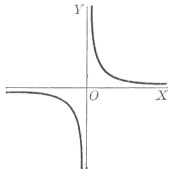# Roots Relation

Algebra Level 5$\large 24x^4 - 15x^3 + 1$

Let $a,b,c,d$ be the roots of the polynomial above. If $(abc)^3+(abd)^3+(acd)^3+(bcd)^3 = \frac pq$ for coprime positive integers $p,q$, find the value of $p+q$.

###### Image Credit: Wikimedia William Anthony Granville.
×

Problem Loading...

Note Loading...

Set Loading...